# Directed acyclic graph

(diff) ← Older revision | Latest revision (diff) | Newer revision → (diff)
A directed acyclic graph (DAG) is a directed graph that contains no cycles. DAGs arise in a natural way in modelling situations in which, in some sense, going "forward" is sometimes possible but going "backward" is definitely not, so that if$v$ is reachable from$u$, we know that$u$ is not reachable from$v$ (unless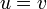$u = v$). An example is given by Water Park from CCC 2007. In the absence of mechanical intervention, water always flows from high altitudes to low altitudes, so that, when travelling along the waterslides, one can never arrive at the same spot twice. If we create a graph that has a vertex for each marked point in the water park and a directed edge from$u$ to$v$ whenever there is a water slide from$u$ to$v$, this graph will be acyclic, and hence a DAG. DAGs do not necessarily resemble undirected acyclic graphs (that is, forests) in form or in their properties; there may be multiple paths between a given pair of nodes.
2. We define our partial ordering as follows: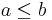$a \leq b$ in the ordering if and only if there exists a path from$u$ to$v$, where$u$ is the vertex in the DAG labelled with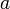$a$ and$v$ is the vertex in the DAG labelled with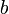$b$.
The ordering thus defined is reflexive, since every vertex is reachable from itself. It is antisymmetric, because if$u \leq v$ and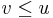$v \leq u$, and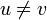$u \neq v$, then we have a cycle that contains$a$ and$b$, a contradiction. And it is transitive because reachability is transitive; if there are paths from$u$ to$v$ and from$v$ to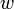$w$ then we can concatenate them to obtain a path from$u$ to$w$. So the ordering we have defined is a partial ordering.